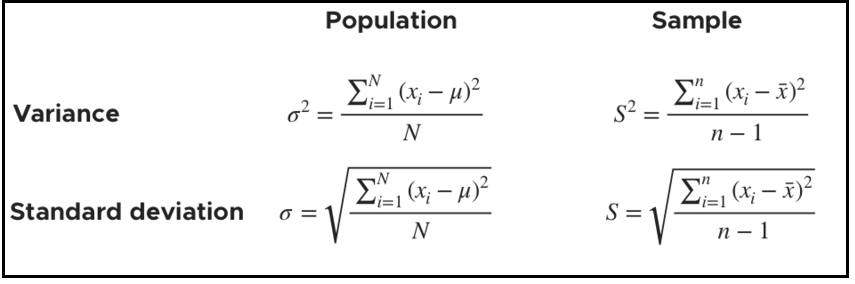Checkout JEE MAINS 2022 Question Paper Analysis : Checkout JEE MAINS 2022 Question Paper Analysis :

# Variance and Standard Deviation

Variance and Standard Deviation are the two important measurements in statistics. Variance is a measure of how data points vary from the mean, whereas standard deviation is the measure of the distribution of statistical data. The basic difference between both is standard deviation is represented in the same units as the mean of data, while the variance is represented in squared units. Let us learn here more about both the measurements with their definitions, formulas along with an example.

## Variance

According to layman’s words, the variance is a measure of how far a set of data are dispersed out from their mean or average value. It is denoted as ‘σ2’.

### Properties of Variance

• It is always non-negative since each term in the variance sum is squared and therefore the result is either positive or zero.
• Variance always has squared units. For example, the variance of a set of weights estimated in kilograms will be given in kg squared. Since the population variance is squared, we cannot compare it directly with the mean or the data themselves.

## Standard Deviation

The spread of statistical data is measured by the standard deviation. Distribution measures the deviation of data from its mean or average position. The degree of dispersion is computed by the method of estimating the deviation of data points. It is denoted by the symbol, ‘σ’.

### Properties of Standard Deviation

• It describes the square root of the mean of the squares of all values in a data set and is also called the root-mean-square deviation.
• The smallest value of the standard deviation is 0 since it cannot be negative.
• When the data values of a group are similar, then the standard deviation will be very low or close to zero. But when the data values vary with each other, then the standard variation is high or far from zero.

## Variance and Standard Deviation Formula

As discussed, the variance of the data set is the average square distance between the mean value and each data value. And standard deviation defines the spread of data values around the mean.The formulas for the variance and the standard deviation for both population and sample data set are given below:

Variance Formula:

The population variance formula is given by:

$$\begin{array}{l}\sigma^2 =\frac{1}{N}\sum_{i=1}^{N}(X_i-\mu)^2\end{array}$$

Here,

σ2 = Population variance

N = Number of observations in population

Xi = ith observation in the population

μ = Population mean

The sample variance formula is given as:

$$\begin{array}{l}s^2 =\frac{1}{n-1}\sum_{i=1}^{n}(x_i-\overline{x})^2\end{array}$$

Here,

s2 = Sample variance

n = Number of observations in sample

xi = ith observation in the sample

$$\begin{array}{l}\overline x\end{array}$$
= Sample mean

Standard Deviation Formula

The population standard deviation formula is given as:

$$\begin{array}{l}\sigma =\sqrt{\frac{1}{N}\sum_{i=1}^{N}(X_i-\mu)^2}\end{array}$$

Here,

σ = Population standard deviation

Similarly, the sample standard deviation formula is:

$$\begin{array}{l}s =\sqrt{\frac{1}{n-1}\sum_{i=1}^{n}(x_i-\overline{x})^2}\end{array}$$

Here,

s = Sample standard deviation

### Variance and Standard deviation Relationship

Variance is equal to the average squared deviations from the mean, while standard deviation is the number’s square root. Also, the standard deviation is a square root of variance. Both measures exhibit variability in distribution, but their units vary: Standard deviation is expressed in the same units as the original values, whereas the variance is expressed in squared units.

### Example

Question: If a die is rolled, then find the variance and standard deviation of the possibilities.

Solution: When a die is rolled, the possible outcome will be 6. So the sample space, n = 6 and the data set = { 1;2;3;4;5;6}.

To find the variance, first, we need to calculate the mean of the data set.

Mean, x̅ = (1+2+3+4+5+6)/6 = 3.5

We can put the value of data and mean in the formula to get;

σ2 = Σ (xi – x̅)2/n

σ2 = ⅙ (6.25+2.25+0.25+0.25+2.25+6.25)

σ2 = 2.917

Now, the standard deviation,σ = √2.917 = 1.708

Test your knowledge on Variance And Standard Deviation